# How do you make mu(μ) and nu(ν) symbol in LaTeX?

Belonging to the Greek alphabet, the symbols mu(μ) and nu(ν) are frequently used in mathematics and physics.

There are several ways to use these symbols in LaTeX, all of which we will discuss in this tutorial.

## Use mu(μ) symbol in LaTeX

LaTeX provides a default command to print the mu symbol in a LaTeX document, which is \mu.

This command is used in math mode so if you want to use this symbol in inline mode you have to use single dollar quotations $\mu$.

\documentclass{article}
\usepackage{amsmath} % for \longrightarrow
\begin{document}
$\verb|\mu|\longrightarrow \mu$
$\textbf{m}=\frac{1}{\mu_0}\textbf{B}_r V$
$(\exists\mu^2)(\forall f^\prime)$
$\sum^n_{i=1}\frac{1}{\mu_i}dN_i$
$1\mu m \quad 2\mu m \quad 3\mu m$
\end{document}

Output :### Non-italic mu symbol in text mode

In the example above you can see the output of the \mu command is shown in italic font.

If you want to use the non-italic mu symbol in text mode, LaTeX provides a default command called \textmu.

This command allows you to print this symbol in a LaTeX document in a non-Italian font.

\documentclass{article}
\usepackage{amsmath} % for \longrightarrow
\begin{document}
\verb|\mu|$\longleftrightarrow \mu$\$4pt] \verb|\textmu|\longrightarrow \textmu\\[4pt] 1\textmu m 2\textmu m 3\textmu m\\[4pt] Write \textmu\;in text mode \end{document} Output :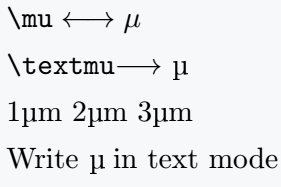### Non-italic mu symbol in math mode You need the upgreek package to use the non-italic mu symbol in latex math mode. This package provides a command called \upmu. With this command, you can print the symbol in the document. You can also use the \muup command included in the txfonts package. There are slight differences between the output of these two commands. So you can use either one as per your need. \documentclass{article} \usepackage{amsmath} % for \longrightarrow \usepackage{upgreek} % for \upmu \usepackage{txfonts} % for \muup \begin{document} \[ \verb|\mu|\longrightarrow\mu \quad\verb|[Default]|$
$\verb|\upmu|\longrightarrow\upmu \quad\verb|[upgreek]|$
$\verb|\muup|\longrightarrow\muup \quad\verb|[txfonts]|$
$(\upmu_0,\upmu_1,\dots,\upmu_{(n-1)},\upmu_n)\quad\verb|[upgreek]|$
$\muup(E)+\muup(\O) \quad\verb|[txfonts]|$
$\sum^\infty_{n=1}\upmu(E_k) \quad\verb|[upgreek]|$
\end{document}

Output :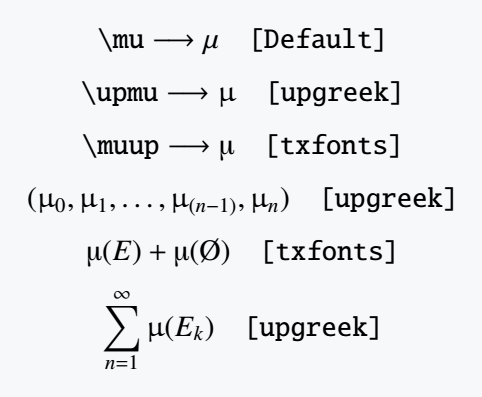## Use nu(ν) symbol in LaTeX

To print the nu symbol in a latex document you need to use the \nu command. This is LaTeX’s default command.

To use this commode in inline mode you must use the single dollar quotation $\nu$.

\documentclass{article}
\usepackage{amsmath} % for \longrightarrow
\begin{document}
$\verb|\nu|\longrightarrow\nu$
$\nu =\frac{\mu}{\rho}$
$\tilde{\nu}=\frac{1}{\lambda}$
$(\nu_0,\nu_1,\dots,\nu_{(n-1)},\nu_n$
$\nu^{\text{Hencky}}=-\frac{\ln\lambda_{\text{trans}}}{\ln\lambda_{\text{axial}}}$
\end{document}

Output :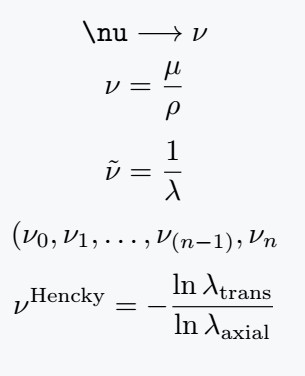### Upright nu symbol in LaTeX

First of all, you need to use the upgreek package for the upright nu symbol. You can print this symbol in a latex document with the \upnu command included in this package.

\documentclass{article}
\usepackage{amsmath} % for \longrightarrow
\usepackage{upgreek} % for \upnu
\begin{document}
$\verb|\nu|\longrightarrow\nu$
$\verb|\upnu|\longrightarrow\upnu$
$\tilde{\upnu}=\frac{1}{\lambda}$
$(\upnu_0,\upnu_1,\dots,\upnu_{(n-1)},\upnu_n$
\end{document}

Output :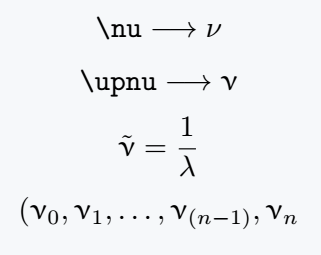## Bold mu and nu symbol

The best package to bold a symbol is bm. This package provides you with one command, which is \bm{}.

With this command, you can print any symbol in bold font.

\documentclass{article}
\usepackage{amsmath} % for \longrightarrow
\usepackage{upgreek} % for \upnu
\usepackage{bm} % for bold symbol
\begin{document}
$\verb|\mu|\longrightarrow\mu$
$\verb|\bm{\mu}|\longrightarrow\bm{\mu}$
$\verb|\upmu|\longrightarrow\upmu$
$\verb|\bm{\upmu}|\longrightarrow\bm{\upmu}$
$\verb|\nu|\longrightarrow\nu$
$\verb|\bm{\nu}|\longrightarrow\bm{\nu}$
$\verb|\upnu|\longrightarrow\upnu$
$\verb|\bm{\upnu}|\longrightarrow\bm{\upnu}$
\end{document}

Output :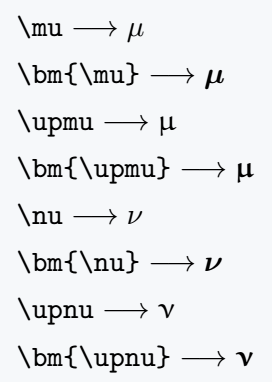#### Md Jidan Mondal

LaTeX expert with over 10 years of experience in document preparation and typesetting. Specializes in creating professional documents, reports, and presentations using LaTeX.

### 2 thoughts on “How do you make mu(μ) and nu(ν) symbol in LaTeX?”

1.You are the best!

1.Thank You.